# Connectivity of Graph

MathematicsComputer EngineeringMCA

Whether it is possible to traverse a graph from one vertex to another is determined by how a graph is connected. Connectivity is a basic concept in Graph Theory. Connectivity defines whether a graph is connected or disconnected. It has subtopics based on edge and vertex, known as edge connectivity and vertex connectivity. Let us discuss them in detail.

## Connectivity

A graph is said to be connected if there is a path between every pair of vertex. From every vertex to any other vertex, there should be some path to traverse. That is called the connectivity of a graph. A graph with multiple disconnected vertices and edges is said to be disconnected.

## Example 1

In the following graph, it is possible to travel from one vertex to any other vertex. For example, one can traverse from vertex 'a' to vertex 'e' using the path 'a-b-e'.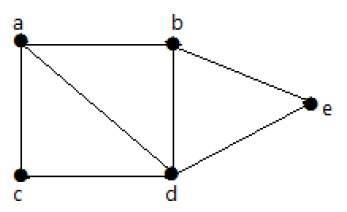## Example 2

In the following example, traversing from vertex 'a' to vertex 'f' is not possible because there is no path between them directly or indirectly. Hence it is a disconnected graph.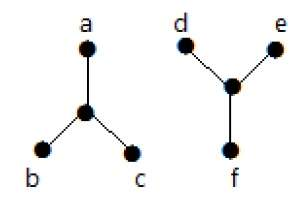## Connectivity Types

Graph Connectivity can be classified broadly into two categories −

• Edge Connectivity

• Vertex Connectivity

## Edge Connectivity

Let 'G' be a connected graph. The minimum number of edges whose removal makes 'G' disconnected is called edge connectivity of G.

Notation − λ(G)

In other words, the number of edges in a smallest cut set of G is called the edge connectivity of G.

If 'G' has a cut edge, then λ(G) is 1. (edge connectivity of G.)

## Example

Take a look at the following graph. By removing two minimum edges, the connected graph becomes disconnected. Hence, its edge connectivity (λ(G)) is 2.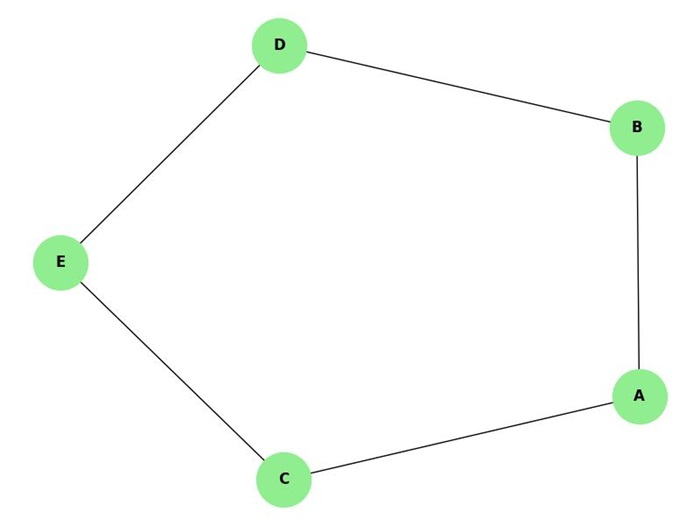Here are the four ways to disconnect the graph by removing two edges −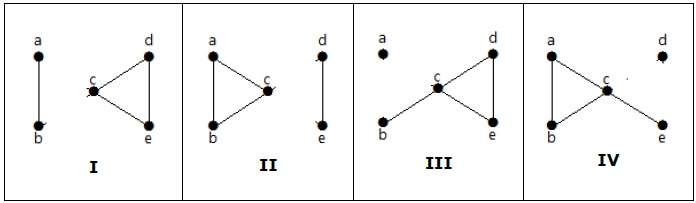## Vertex Connectivity

Let 'G' be a connected graph. The minimum number of vertices whose removal makes 'G' either disconnected or reduces 'G' in to a trivial graph is called its vertex connectivity.

Notation − K(G)

## Example

In the above graph, removing the vertices 'e' and 'i' makes the graph disconnected.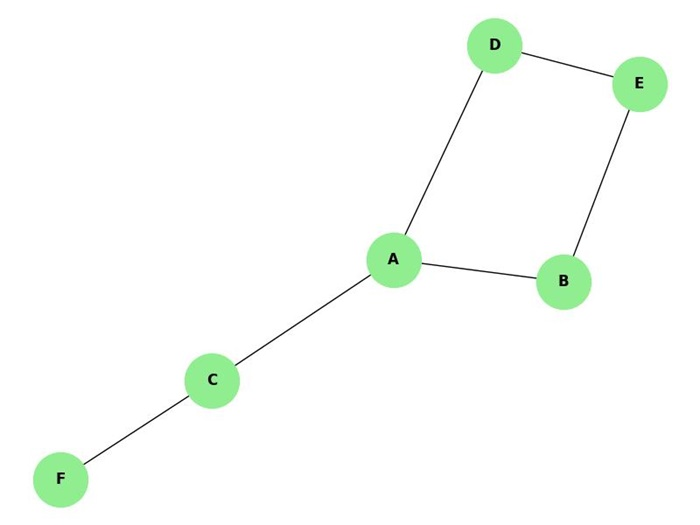If G has a cut vertex, then K(G) = 1.

Notation − For any connected graph G,

K(G) ≤ λ(G) ≤ δ(G)

Vertex connectivity (K(G)), edge connectivity (λ(G)), minimum number of degrees of G(δ(G)).

## Example

Calculate λ(G) and K(G) for the following graph −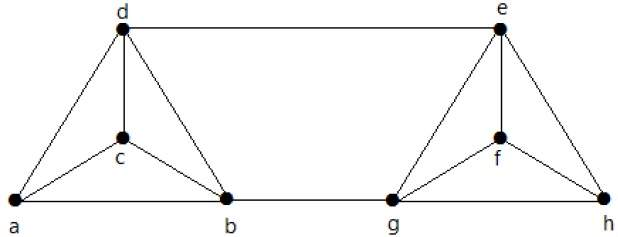Solution

From the graph,

δ(G) = 3

K(G) ≤ λ(G) ≤ δ(G) = 3 (1)

K(G) ≥ 2 (2)

Deleting the edges {d, e} and {b, h}, we can disconnect G.

Therefore,

λ(G) = 2

2 ≤ λ(G) ≤ δ(G) = 2 (3)

From (2) and (3), vertex connectivity K(G) = 2